How Cheenta works to ensure student success?
Explore the Back-Story

# NMTC 2023 Stage II - Gauss (Grade 5 & 6) - Problems and Solutions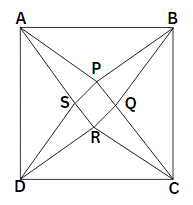###### Problem 1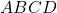is an isosceles trapezium as shown in the figure, in which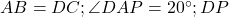is perpendicular to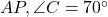;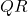is the bisector of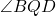and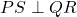.

Calculate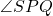and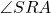.
Justify each of the steps in calculation.

###### Problem 2

Ramanujan is a sixth grade student. His mathematics teacher gave a problem sheet in maths as home task for the Puja holidays. Ramanujan wants to complete it in 4 days and wants to enjoy the holidays for the remaining 6 days.

On the first day, he worked out one-fifth the number of problems plus 12 more problems.

On the second day, he worked out one-fourth the remaining problems plus 15 more problems.

On the third day, he solved one-third of the remaining problems plus 20 more problems.

The fourth day, he worked out successfully the remaining 60 problems and completed the work.

Find the total number of problems given by the teacher and the number of problems solved by Ramanujan on each day.

###### Problem 3

There are 4 cards and on each card a whole number is written. All the numbers are different from one another. Two girls of grade six, Deepa and Dilruba play a game.

Deepa takes 3 cards at a time leaving a card behind. She multiplies the numbers and gets an answer. In the same way, again, she leaves one different card and selects the other three and multiplies the numbers. She got the answers 480, 560, 420 and 336 .

Now, Dilruba has to find the numbers in each card. Dilruba worked out and found the correct numbers.

What are they? Work out systematically and find the numbers.

###### Problem 4

An angle is divided into 3 equal parts by two straight lines; such lines are called trisectors.is a square. The lines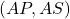are trisectors of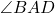. Similarly, we have the trisectors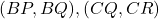and. Prove that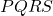is a square.

###### Problem 5

Five squares of different dimensions are arranged in two ways as shown in the following diagrams. The numbers inside each square represents its area in square units.

Calculate the perimeterand the corresponding perimeter of figure 2 . Are they same? If they are different, which is greater?

###### Problem 6

(i) In a book, a problem on fractions is given as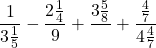The denominator of the third term is not printed. The answer is given to be 2 . What is the missing denominator? Let it be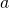.

(ii) Simplify: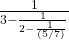. Let the answer be of the form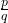where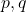have no common factors. Let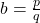.

(iii) Find the value of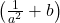###### Problem 7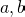are two integers. Find all pairsand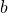such that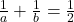. Arrive at your result logically.

###### Problem 8

A train starts from a station A and travels with constant speed up to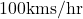. After some time, there appeared a problem in the engine and so the train proceeds withth of the original speed and arrives at Station B, late by 90 minutes. Had the problem in the engine occurred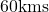further on, then the train would have reached 15 minutes sooner. Find the original speed of the train and distance between stationsand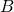.

###### Problem 1is an isosceles trapezium as shown in the figure, in whichis perpendicular to;is the bisector ofand.

Calculateand.
Justify each of the steps in calculation.

###### Problem 2

Ramanujan is a sixth grade student. His mathematics teacher gave a problem sheet in maths as home task for the Puja holidays. Ramanujan wants to complete it in 4 days and wants to enjoy the holidays for the remaining 6 days.

On the first day, he worked out one-fifth the number of problems plus 12 more problems.

On the second day, he worked out one-fourth the remaining problems plus 15 more problems.

On the third day, he solved one-third of the remaining problems plus 20 more problems.

The fourth day, he worked out successfully the remaining 60 problems and completed the work.

Find the total number of problems given by the teacher and the number of problems solved by Ramanujan on each day.

###### Problem 3

There are 4 cards and on each card a whole number is written. All the numbers are different from one another. Two girls of grade six, Deepa and Dilruba play a game.

Deepa takes 3 cards at a time leaving a card behind. She multiplies the numbers and gets an answer. In the same way, again, she leaves one different card and selects the other three and multiplies the numbers. She got the answers 480, 560, 420 and 336 .

Now, Dilruba has to find the numbers in each card. Dilruba worked out and found the correct numbers.

What are they? Work out systematically and find the numbers.

###### Problem 4

An angle is divided into 3 equal parts by two straight lines; such lines are called trisectors.is a square. The linesare trisectors of. Similarly, we have the trisectorsand. Prove thatis a square.

###### Problem 5

Five squares of different dimensions are arranged in two ways as shown in the following diagrams. The numbers inside each square represents its area in square units.

Calculate the perimeterand the corresponding perimeter of figure 2 . Are they same? If they are different, which is greater?

###### Problem 6

(i) In a book, a problem on fractions is given asThe denominator of the third term is not printed. The answer is given to be 2 . What is the missing denominator? Let it be.

(ii) Simplify:. Let the answer be of the formwherehave no common factors. Let.

(iii) Find the value of###### Problem 7are two integers. Find all pairsandsuch that. Arrive at your result logically.

###### Problem 8

A train starts from a station A and travels with constant speed up to. After some time, there appeared a problem in the engine and so the train proceeds withth of the original speed and arrives at Station B, late by 90 minutes. Had the problem in the engine occurredfurther on, then the train would have reached 15 minutes sooner. Find the original speed of the train and distance between stationsand.

This site uses Akismet to reduce spam. Learn how your comment data is processed.

### Knowledge Partner